# Clustering

Agglomerative hierarchical clustering is a distance based method to find structure in data sets.

In our case, clusters are built from genes (hybridizations), in such a way that genes (hybridizations) with highest similarity - or reverse: smallest distance - are grouped togehter into clusters.

Aim is to organize all gene-vectors (hybridfization-vectors) into a binary tree (dendrogram).

The clustering process:

Initialize a distance matrix by computing all vector-vector distances between all objects (genes/hybridizations).
Iteratively compute:
• find pair of vectors with smallest distance
• combine two closest vectors - single genes or average vectors from already assembed subclusters - into a new "subcluster" and remove original objects
• recompute all distances between new "subcluster" and all others
Repeat this iteration until only one cluster - containing all objects - is left over.

The clustering process is memory and time intensive. The distance matrix for 10000 genes will require ~400MByte of RAM, for 30000 genes ~3 GigaByte are required.
Clustering 1000 genes is done in a view seconds, 10000 genes require several minutes, 30000 genes hours.

A key point in clustering is: how to measure distances/similarieties between the idividual data vectors.

Different distance measures may generate quite different cluster results.
Thus, it is important to select the distance metric wich is most adeqate for the specific dataset and question.

See below for distances metrices supported by SUMO

See a very simple example illustrating the overall clustering process.
(6 "genes" with two expression values each, applying Manhattan distance metric and average linkage)

## Hierarchical clustering with SUMO

Select Cluster from the main menu: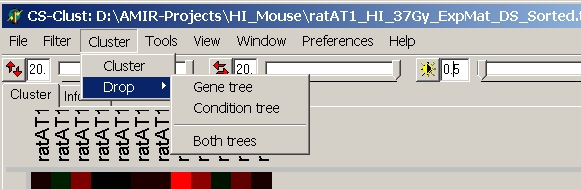## Cluster

The cluster dialog opens up: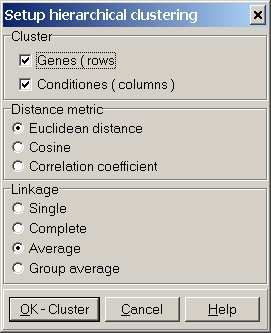Define the parameters used for clustering:

### Cluster

Select to cluster Genes and/or Conditions (=Hybridizations).
Clustering may be performed in one go, or you can first cluster genes, then cluster conditions with different distance metric or linkage.

### Distance metric

Several distance metrics may be selected. See below for more details about the metrics.

At present three linkage methods may be selected (Group average does not work yet). See below for more details about the metrics.

### OK-Cluster button

Run clustering algorithm with specified parameters.

A progress indicator box will show up.The lower progress indicator bar shows the main processing steps:

• gene distance calculation
• gene clustering
• condition distance calculation
• condition clustering

The upper progress indicator bar shows the progress of each of the (up-to) four steps.

After clustering, the heatmap view will update and show the computed trees: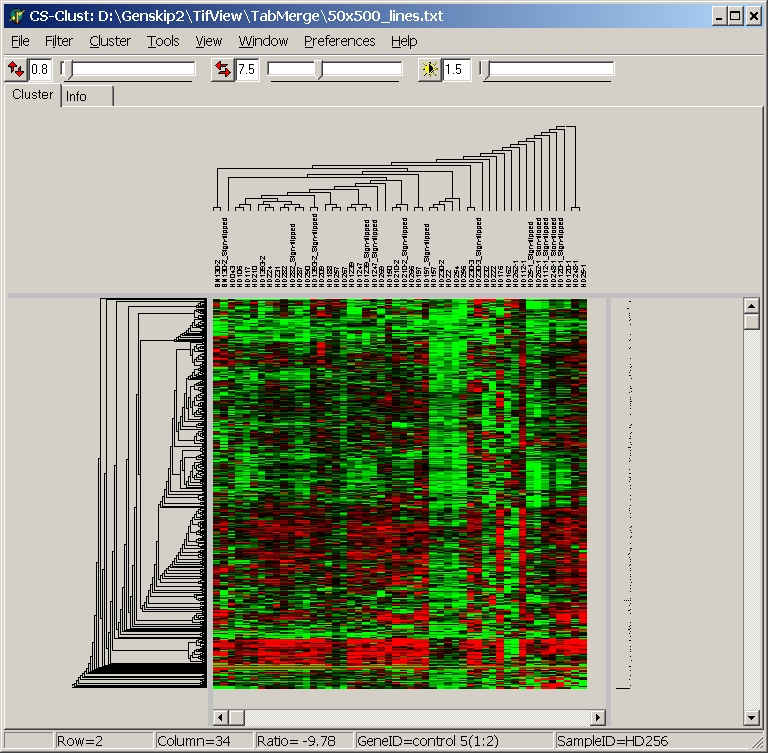## Distance Metric

A key in clustering is: how to measure simlarity / distance between data vectors (gene's expression profiles over multiple conditions or condition's profiles over multiple genes).

Therefore, one can define the mathematical concept of a metric.
A metric on a set is a function (the distance function d(x,y) between two vectors x and y) with the fundamental properties:

 1 d(x,y) ≥ 0 non negativity 2 d(x,y) = d(y,x) symmetry 3 d(x,x) = 0 identity 4 d(x,y) = 0, if and only if x = y definiteness 5 d(x,z) ≤ d(x,y) + d(y,z) triangle inequation

Now you can freely define a function fulfilling the above properties as a distance measure.

Commonly used methods are:

Different distance metrics will measure different properties of the data.
As a result, clustering with different distance metrics may generate completely different trees.
Which distance metric to use, depends on the question you want to be answered by your clustering.

### Minkowski distance

The Minkowski distance is a generalized distance metric in Euclidean space.

Assume two vectors P,Q with dimension n: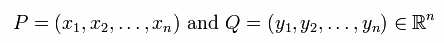The Minkowski distance of order p is defined as: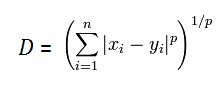Most commonly used are order p= 1,2 and infinite.

 Lets look at a very simple example in two dimensional space (n=2):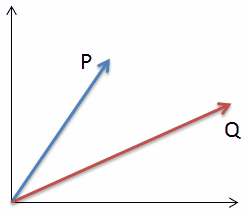Manhattan distance (p=1):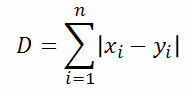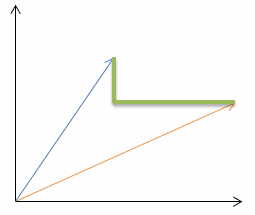Euclidean distance (p=2):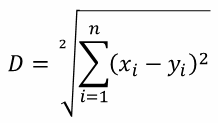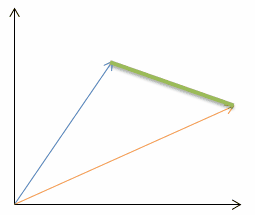Chebyshev distance (p=infinite):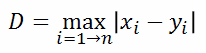### Distances metrices similar to Manhattan distance:

#### Gower distance

A variant of the Manhatton distance, divided the vector dimension: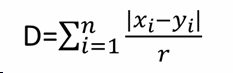with r = n

### Lorentzian distance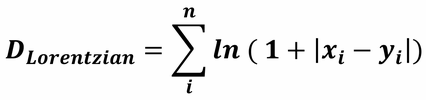### Canberra distance

A variant of the Manhattan distance, where each component is normalized to its "length":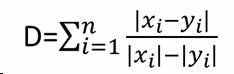### Bray-Curtis / Sorensen / Soergel / Czekanowski distance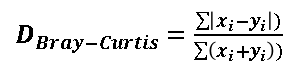### Intersection distance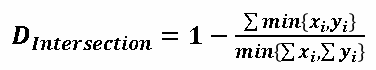### Penrose shape distance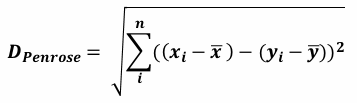### Meehl distance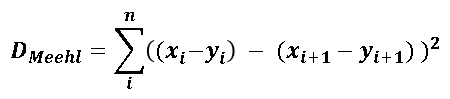### Hellinger distance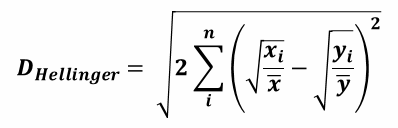### Clark distance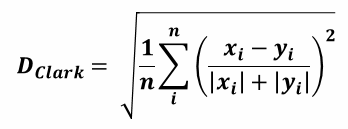### Correlation distance

A correlation coefficient is probable more familiar in (linear) regression.

Lets take our two vectors (P,Q), and plot the components (x1,y1), (x2,y2), (x3,y3), ... in a 2-d scatter plot: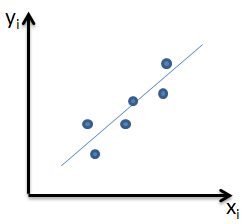If the shape of the data vectors is similar, the data points should be closely scattered around a line (linear regression).
E.g. the two genes (P and Q) are always expressed / regulated in the same way (up / down) for each single hybridisation.

Now we can test whether there is a certain denpendance (=correlation) between the two data vectors:

#### Pearson correlation coefficient

We can test how good the components are lying on a line by computing the Pearson correlation coefficient: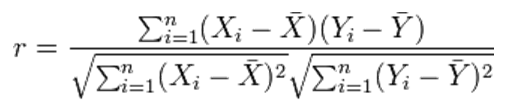with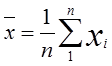The values for r range from -1 ≤ r ≤ 1:
 1 = identical shape of vectors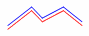0 = no similarity in vector's shape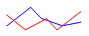-1 = complete opposite shape of the vectors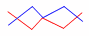To transform the Pearson Correlation coefficient to a distance we compute:

dPC = 1 - r

• 0 = identical shape of vectors
• 1 = no similarity in vector's shape
• 2 = complete opposite shape of the vectors
All three examples view pairs of nicely correlating vectors: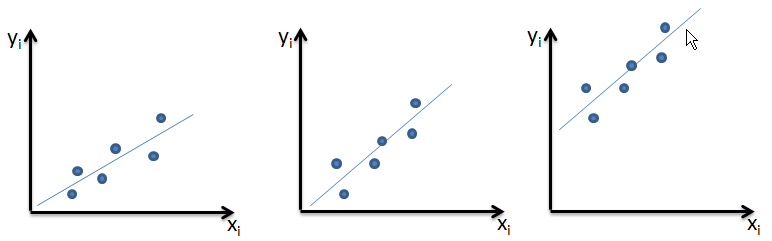also:
• slope af the regresson line might be different from 1
• absolute values between the vectors might be quite different

The picture illustrates in more details the result of pearson correlation (taken from
Wikipedia):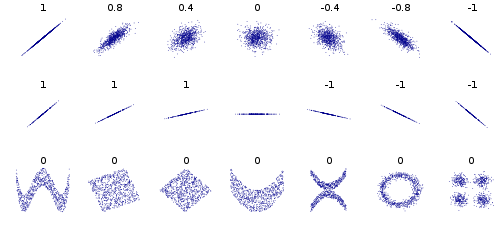Several sets of (x, y) points, with the correlation coefficient of x and y for each set. Note that the correlation reflects the non-linearity and direction of a linear relationship (top row), but not the slope of that relationship (middle), nor many aspects of nonlinear relationships (bottom). N.B.: the figure in the center has a slope of 0 but in that case the correlation coefficient is undefined because the variance of Y is zero.

#### Spearman's rank correlation coefficient

As discussed above, Pearson correlation can only give r=1 if the components of both vectors are related by a linear function.
If the data vectors form a perfect e.g. banana or sigmoid shaped scatterplot, the Pearson correlation coefficient will return values r < 1.

Spearman's rank correlation is a non parametric measure of statistical dependence and tests whether the relation between our two vectors may be described by a monotonic function.

Data vectors are transformed into ranked variables.

Assume two vectors:
 P 4 2 10 6 8 Q 5 3 42 20 40
Pearson correlation coefficient: r ~ 0.81

Lets rank both vectors (i.e. sort by magnitude):
 P 4 2 10 6 8 Ranks(P) 2 1 5 3 4 Q 5 3 42 20 40 Ranks(Q) 2 1 5 3 4

With the new rank vectors we compute the Pearson correlation coefficient:
 Ranks(P) 2 1 5 3 4 Ranks(Q) 2 1 5 3 4
Spearman's rank correlation coefficient = 1

In the present version, SUMO does not take care of ties (= identical numerical value for multiple vector components), as they are not very likely to appear in quasi continuous floating point expression intensities or ratios.

#### Kendall's Tau correlation

Kendall Tau correlation coefficient measures even more generalized the association between two datasets.
The non-parametric tests measures the rank correlation, which means the similarity in the order of the data after ranking (=sorting).

Assume two data vectors:
 P 4 2 10 6 8 Q 5 20 42 40 3

Lets rank both vectors:
 P 4 2 10 6 8 Ranks(P) 2 1 5 3 4 Q 5 20 42 40 3 Ranks(Q) 2 3 5 4 1

Now we analyze the ranks:
 A B C D E Ranks(P) 2 1 5 3 4 Ranks(Q) 2 3 5 4 1

Lets analyze each possible pair of data values from vector P and Q.
• Count the pairs where the order of values is identical (concordant pairs, nc )
• Count the pairs where the order of values is inversed (disconcordant pairs, nd )
 Pair Data concordant dis-concordant A-B 2 > 12 < 3 X A-C 2 < 52 < 5 X A-D 2 < 32 < 4 X A-E 2 < 42 > 1 X B-C 1 < 53 < 5 X B-D 1 < 33 < 4 X B-E 1 < 43 > 1 X C-D 5 > 35 < 4 X C-E 5 > 45 < 1 X D-E 3 < 44 > 1 X Sum 6 4

4 data pairs have inversed order, thus Kendall tau distance is 4.
Normalized to the number of pairs, Kendall tau distance is 0.4 indicating low association in the rankings.

Similarly we compute the correlation coefficient: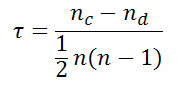with nc=number of concordant pairs nd=number of disconcordant pairs) n=dimension of data vectors

In the example τ = 0.44, indicating nearly no correlation.

In the present version, SUMO does not take care of ties (= identical numerical value for multiple vector components), as they are not very likely to appear in quasi continuous floating point expression intensities or ratios.

#### Cosinus distance

The Cosinus distance measures the angle between the two vectors P and Q.
The formula and result are very similar to correlation distance.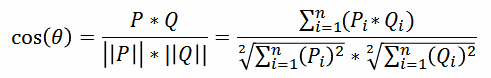#### "Dot product"

Minkowski distances are sensitive to the length of the vecotors, correlation distances to the angle between the vectors.

An alternative measure could combine both informations.

The dot product between two vectors is defined as: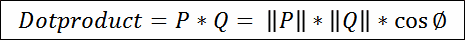Unfortunately, the dotproduct does not fulfill the requirements of a distance metric.
The Dotproduct between two identical vectors is >0, the dotproduct between two orthogonak vectors = 0.

But we can use a modified "dotproduct", where we replace the cosine function with a sine: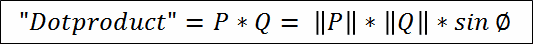### Binary distances

Special metrices for binary data - a bit unconventional for gene expression data.
For these metrics, data should contain only "1" and "0" vlaues - binary data.
Continuous data vs. binary distance metrices Before clustering with binary distrance, SUMO checks the data:
• All data are binary, i.e. only "1" and "0": data are diretly clustered
• anything else - perform a temporary binarisation.
Define a threshold value - any data cell >= Threshold will be transformed to "1"
- all other data cells will be transformed to "0"
Clustering is now performed with temporary binarized data
After clustering, the "normal" continuous date are restored.
It may be recommended to permanently transform continuous expression data into binary (1 or 0) values before clustering data when using binary distance.
Use SUMO Main menu | Adjust data | Data transformation | Binarization

Assume two gene / condition vectors xi and xj with p elements (in the example p=12 elements):

xi = ( 1,1,0,1,0,0,1,1,0,1,0,1 )
xj = ( 1,0,1,1,0,1,1,0,0,0,1,1 )

Now we can count:

 n11 number of common existing elements in both vectors ("1" - "1").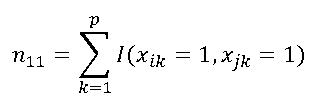In the example: xi = ( 1,1,0,1,0,0,1,1,0,1,0,1 ) xj = ( 1,0,1,1,0,1,1,0,0,0,1,1 ) n11= 4 n00 number of common missing elements in both vectors ("0" - "0").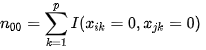In the example: xi = ( 1,1,0,1,0,0,1,1,0,1,0,1 ) xj = ( 1,0,1,1,0,1,1,0,0,0,1,1) n00= 2 n10 number of elements only exisitng in first vector ("1" - "0") .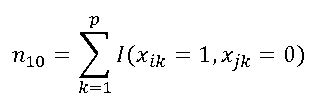In the example: xi = ( 1,1,0,1,0,0,1,1,0,1,0,1 ) xj = ( 1,0,1,1,0,1,1,0,0,0,1,1 ) n10= 3 n01 number of elements only exisitng in second vector ("0" - "1")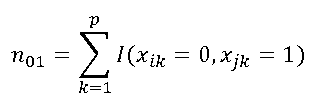In the example: xi = ( 1,1,0,1,0,0,1,1,0,1,0,1 ) xj = ( 1,0,1,1,0,1,1,0,0,0,1,1 ) n01= 3

With the count numbers n11, n00, n01, n10 we can compute several similarity / distance measures:

#### Jaccard distance

Jaccard Index (similarity):

JI = n11 / ( n11 + n10 + n01 )

here: JI = 4 / (4+3+3) = 0.4

Jaccard distance:

DJaccard = 1 - JI

Here: DJaccard = 1-0.4 = 0.6

#### Hamming distance

Count the number of components different in both vectors, but not Zero in both:

DHamming = n10 + n01

Here:DHamming = 3+3 = 6

#### Simple Matching Distance

Normalized Hamming distance.

DSimpleMatching = ( n10 + n01 ) / p
Here: DSimpleMatching = ( 3+3 ) / 12 = 0.5

#### Russel-Rao

Normalized count of positive matches.

DRussel-Rao = 1 - n11 / p
Here: DRussel-Rao = 1 - 4/12 = 0.333
Russel-Rao= DIce / 2

#### Dice

Normalized count of positive matches.
Dice = Russel-Rao*2

DDice = 2 * n11 / p
Here: DDice = 2- 2*4/12 = 1.333

#### Tanimoto

Normalized count of positive matches.

DTanimoto = 1 - (n11 + n00) / (n00 + 2*(n01+n10) + n11)
Here: DTanimoto = 1 - (4+2)/(2+2*(3+3)+4) = 1 - 6/18 = 0.666

#### Braun

DBraun = 1 - n11 / max((n11++n01),(n11++n19))
Here: DBraun = 1 - 4/7 = 0.5667

#### Kulczynski

Kulczynski-Similarity = n11 / (n01+n10)

DKulczynski = 1 - (/p)
Here: DKulczynski = 1 - 4/6 = 0.666

#### Simpson

Simpson-similarity = Min (n11+n01),(n11+n10

DSimpson = 1 - Simpson-similarity

Here: DSimpson = 1 - 4/7 = 0.429

#### Kappa

Kappa-similarity = 1 / (1 + n*((n01+n10)/(2*(n00+n11)-(n01*n10))))

DKappa = (1 - Kappa-similarity) / 2

Here: DKappa = (1 - (6-6/12) = 0.5

#### Hamann

Hamann-similarity = Min ((n11+n00) - (n01+n10)) / p

DHamann = (1 - Hamann-similarity) / 2

Here: DHamann = (1 - (6-6/12) = 0.5

#### Sneath

Ochiai-similarity = n11 / sqrt ((11+(n01)+(n11+n10))

DOchiai = 1 - Ochiai-similarity

Here: DSneath = 1 - 4/sqrt(7*7) = 0.429

#### Sneath

Sneath-similarity = n11 / (11+(n01+n10))

DSneath = 1 - Sneath-similarity

Here: DSneath = 1 - 4/(4+3+3= = 0.6

#### Yule

Yule-similarity = (n11*n00) - (n01*n10)) / (n11*n11) + (n01*n10))
DYule = (1 - Yule-similarity) / 2

Here: DYule = 1 - (4*2 - 3*3) / (4*2 + 3*3) / 2 = 1 - (-1/81)/2 = 0.5

#### Pearson Phi coefficent

Or "mean square contingency coefficient" or "Matthews correlation coefficient"
Phi-coefficent = (n11*n00) - (n01*n10)) / sqrt((n11+n01)*(n11+n10)*(n00+n01)*(n00+n10))

Teh Phi coeffcient gibve s the same resutl as the Pearson correlation wit binary vectors.

DPhi = (1 - Phi-coefficent) / 2

Here: DPhi = (1 - (4*2 - 3*3) / sqrt((4+3)*(4+3)*(2+3)*(2+3))) /2 = (1 - 1/sqrt(1225)) / 2 = 0.486

#### Accuracy

Accuracy = (n11+n00) / p

DAccuracy = 1 - Accuracy

Here: DAccuracy = 1 - (4+2)/12 = 0.5

#### F1-score

F1-score = (2*n11 / (2*n11+n10+n01)

DF1-score = 1 - F1-score

Here: DF1-score = 1 - ?8/(8+3+3) = 0.29